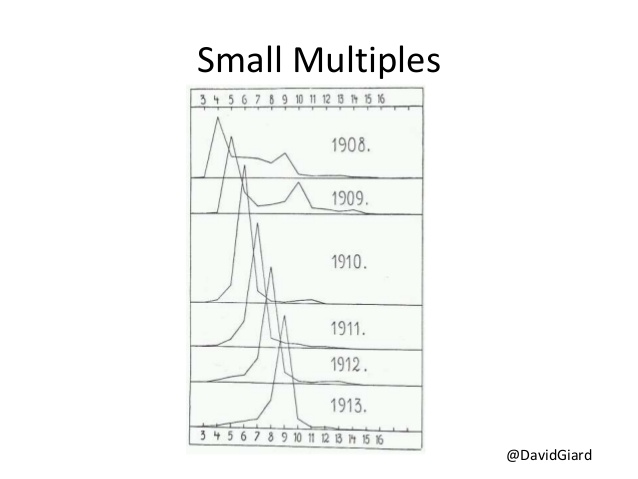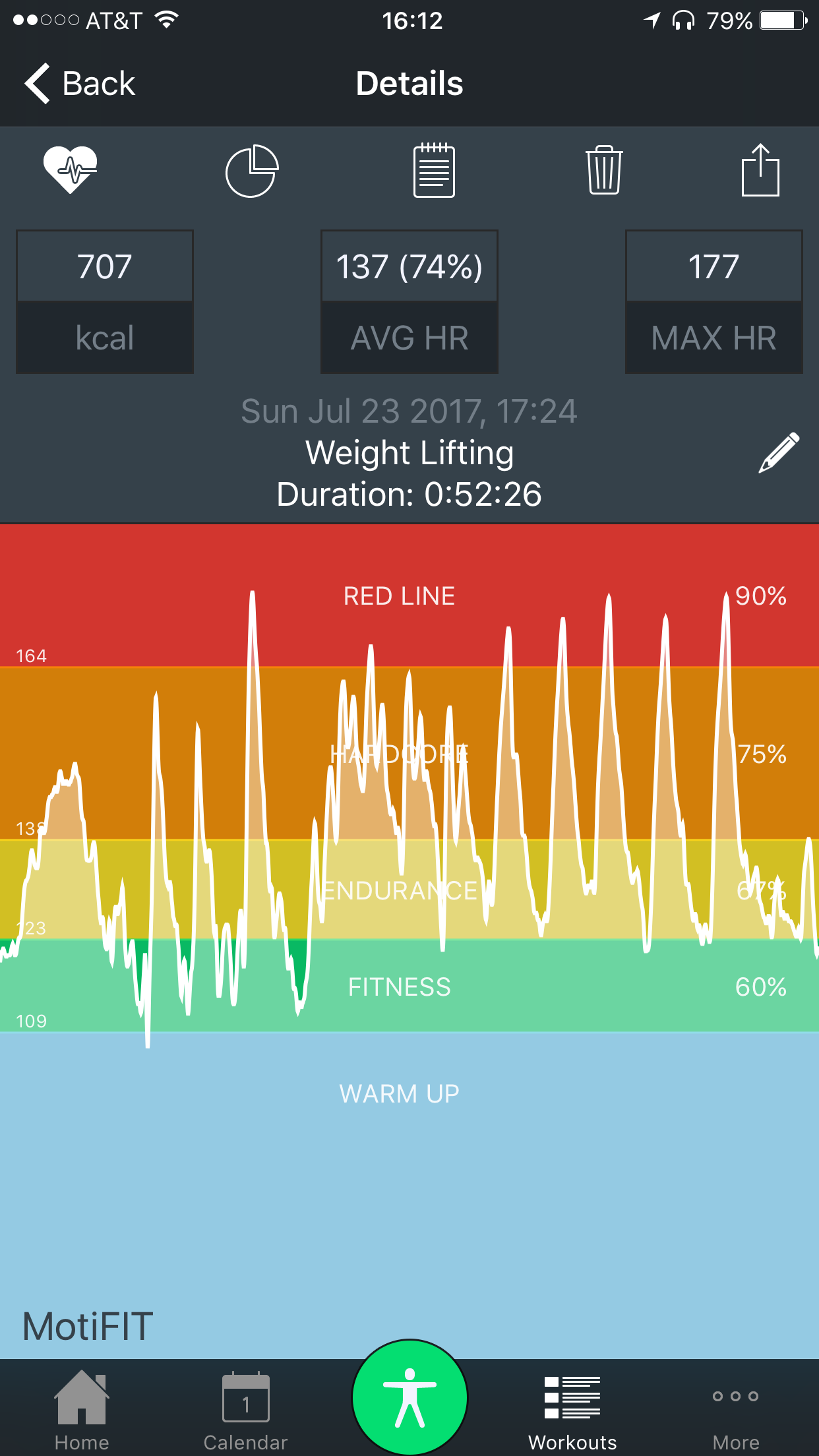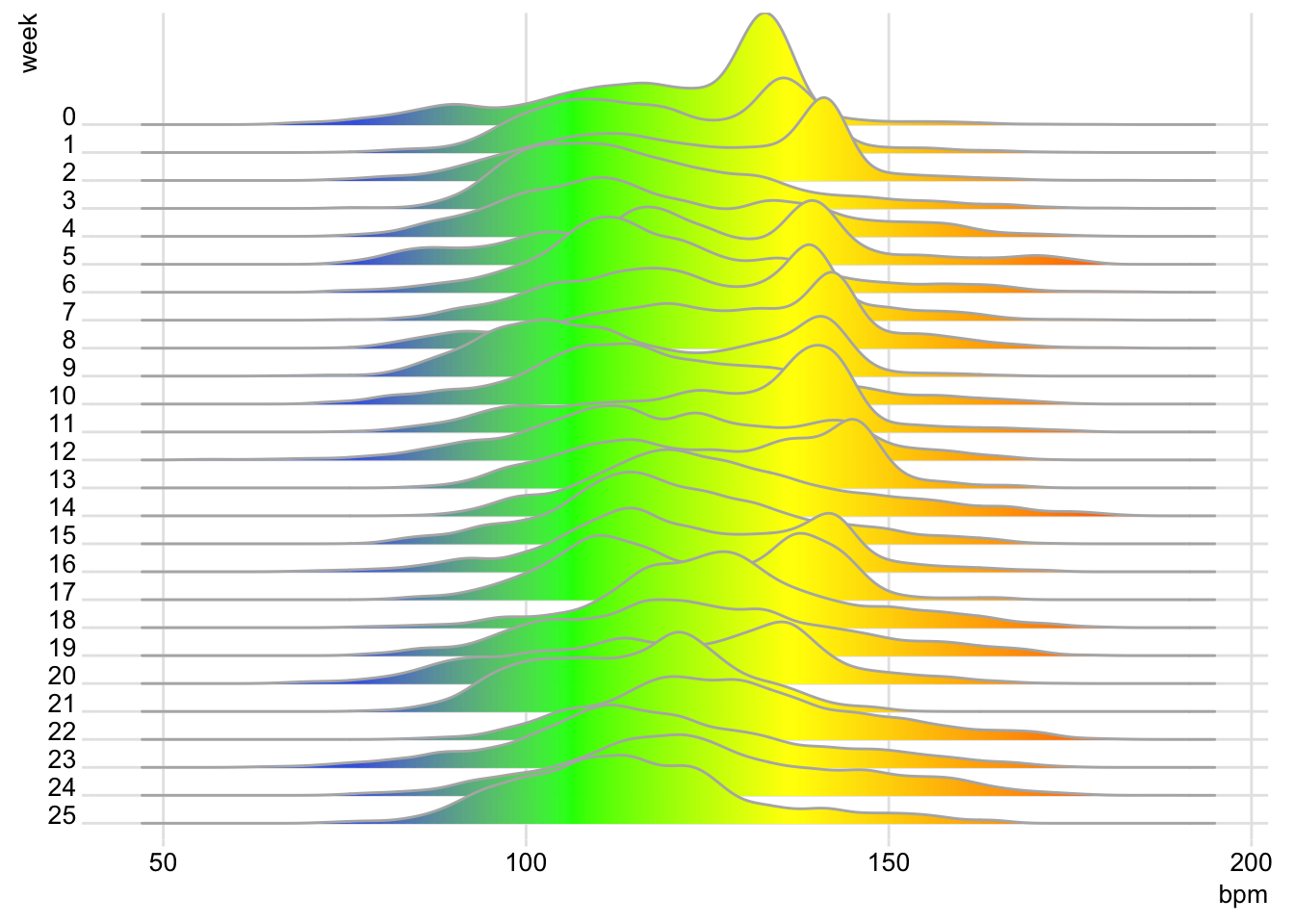The “joy” of plotting heartrate dataThere’s been quite a few posts lately in the R world about the joyplot. I emailed my dataviz instructor from last fall this wonderful post that shows how Congress has become more polarized on one side of the aisle. He pointed out this isn’t as new as everyone thinks it is, as Tufte gives an example of it in one of his books from the early 1900s (this image is from slide 71 of this presentation).

In any event, joyplots are great for comparing values over time. I’ve been wearing a heartrate monitor while at the gym since February, and the MotiFit app lets you export that data to a CSV file. I’ve been saving these files each day after working out, but I haven’t done much with it yet. After gathering 6 months of data, it seemed high time to play around with joyplots!

The first thing I needed to do was combine all the files, which wasn’t as straightforward as I hoped. The MotiFit app exported data in two different formats and two different encodings, so I had to account for this. I wound up guessing the encodings from the readr package, and then combining the date and time columns where necessary.

``````out.file <- data.frame()
file.names <- dir(datadir, pattern = "HeartRateData.+?.csv") # You'll need to set datadir

for (i in 1:length(file.names)){
# exported files are in at least 2 different encodings, so we're going to guess using the guess_encoding function from the readr package
encoding <- guess_encoding(paste(datadir, "/", file.names[i], sep=""), n_max = 1000)
skip = 1,
stringsAsFactors = FALSE,
fileEncoding = toString(encoding[1,1])
)

# exported data has 2 or three columns, so if it's three, we're going to join the date and time fields
if (length(file) > 2) {
temp.date <- file[,1]
temp.time <- trimws(file[,2])
temp.bpm <- trimws(file[,3])

# date format is Wed Feb 8 2017
temp.datetime <- as.POSIXct(paste(temp.date, temp.time, sep=" "), format = "%a %b %d %Y %H:%M")

temp.df <- data.frame(temp.datetime, temp.bpm)
names(temp.df) <- c("timestamp", "bpm")
file <- temp.df
rm(temp.df)
} else {
names(file) <- c("timestamp", "bpm")
file\$timestamp <- as.POSIXct(file\$timestamp)
}

out.file <- rbind(out.file, file)
}``````

Next, I added a week number column. I’ve been lifting since March on the Wendler 5/3/1 program, which has 4-week cycles of varying intensity (including a deload week). So, the intensity of exercise depends primarily on which week it is.

``````library(dplyr)

# Used to calculate week number below
start.date <- min(as.Date(out.file\$timestamp), na.rm=TRUE)

HR <- out.file %>%
mutate(
week = factor(as.numeric(as.Date(timestamp) - start.date) %/% 7, ordered = F),
bpm = as.numeric(bpm)
) %>%
filter(! is.na(week)) %>%
arrange(week)

# Reorder factor so oldest week is at top
HR\$week <- factor(HR\$week, ordered = TRUE, levels = rev(unique(HR\$week)))``````Now that we have the data we need, we can apply the ggjoy! I’m using a custom color scale based on the figures the MotiFit app gives me. To make these look right, however, you need to set the breaks at the midpoint between each value (something I learned in the BMI post).

Here’s the result:

``````library(ggjoy)

bpm.min <- min(HR\$bpm, na.rm = T)
bpm.max <- max(HR\$bpm, na.rm = T)

breaks <- c(
bpm.min,
(bpm.min + 109) / 2,
(109 + 123) / 2,
(123 + 138) / 2,
(138 + 164) / 2,
bpm.max
)

ggplot(
HR,
aes(x = bpm, y = week, height = ..density.., fill = ..x..)
) +
colors = c("royalblue", "royalblue", "green", "yellow", "orange", "red"),
breaks = breaks
) +
geom_joy_gradient(na.rm = TRUE, col = "grey70", scale = 4) +
theme_joy(font_size = 10) +
theme(
legend.position = "none"
)``````The thing that jumps out at me is that you can really identify which weeks I was good about doing cardio work at a target heart rate around 140, and which weeks I wasn’t.

Interactive BMI Chart

I was recently listening to the #WhoIsFat Joe Rogan podcast where comedians Bert Kreischer and Tom Segura had their weight loss challenge weigh-ins. The challenge was for both guys to get out of the “obese” category and into the merely “overweight” category. If one made it and the other didn’t, the loser would pay for a trip to Paris for the winner. If both made it, fellow comedian Ari Shaffir would pay. At the weigh-in, Ari questioned Tom’s height and whether he made it to the overweight BMI. I googled BMI charts to see whether Ari was right. However, the interactivity of the ones I found left something to be desired.

Coincidentally, the same my wife texted me the same day about an impressive BMI (around 50) in a case she was handling. She practices Social Security disability law, and weight, BMI, diabetes, etc. often arise in a disability determination. Between the bad interactive examples I found on Google and my wife’s comments about her case, I decided to make an interactive BMI chart she can use at work.

Since we’re in the US, we’re using imperial units. We’ll go from 100 to 300 pounds in weight and 5’ to 6’6" in height. We’ll use factors instead of continuous variables so we can label height in feet and inches, rather than just inches.

```weights <- seq(from = 100, to = 300, by = 5)
heights <- seq(from = 78, to = 60)

df <- data.frame(height = factor(paste(floor(heights / 12), "'", heights %% 12, "\"", sep=""), labels = rev(paste(floor(heights / 12), "'", heights %% 12, "\"", sep="")), ordered = TRUE))
df\$height <- sort(df\$height, decreasing = TRUE)```

Next, we’ll perform the BMI calculations. That requires conversion from inches to meters and pounds to kilograms. We’ll create a grid of the BMIs that is a text representation of the chart.

```for(x in weights){
bmi.column <- c()
for (y in heights){
# inches to meters
meters <- y * 0.0254
# pounds to kgs
kgs <- x * 0.453592

bmi <- round(kgs / (meters * meters), 1)
bmi.column <- c(bmi.column, bmi)
}
df <- cbind(df, bmi.column)
}
names(df) <- c("height", weights)```

In order to plot the chart, we need to translate the data into key->value pairs that ggplot can use. We’ll calculate the max and min BMIs so we can set our color scales as well.

```library(reshape2)
df <- melt(df, id.vars = c("height"))
names(df) <- c("height", "weight", "bmi")
min.bmi <- min(df\$bmi)
max.bmi <- max(df\$bmi)```

I wanted to create a nice gradient between each BMI level. Here are the BMI levels I used:

• < 18: underweight
• 18-25: normal BMI
• 25-30: overweight
• 30-35: obese
• 35+: morbidly obese
```colors <- c(
"darkgoldenrod",
"goldenrod",
"green",
"yellow",
"red",
"purple",
"purple4"
)
values <- c(
min.bmi,
(min.bmi + 18) / 2,
(18 + 25) / 2,
(25 + 30) / 2,
(30 + 35) / 2,
(35 + 40) / 2,
max.bmi
)
breaks <- c(
min.bmi,
(min.bmi + 18) / 2,
(18 + 25) / 2,
(25 + 30) / 2,
(30 + 35) / 2,
40,
max.bmi
)
labels <- c(
"",
"Underweight",
"Ideal",
"Overweight",
"Obese",
"Morbidly Obese",
""
)```

All the prep work is done now. Let’s plot! Note that the text is commented out. I found that the mouseover brushing didn’t work as well with the labels printed, so I took them out.

```library(ggplot2)
library(scales)
gg <- ggplot(df, aes(x = weight, y = height)) +
geom_raster(aes(fill = bmi), interpolate = TRUE) +
colors = colors,
guide = "colorbar",
values = rescale(values, to = c(0,1),
from = range(df\$bmi)
),
labels = labels,
breaks = breaks
) +
#geom_text(aes(label = bmi), size = 3) +
xlab("weight") +
scale_x_discrete(breaks = seq(from = 100, to = 300, by = 25))

library(plotly)
(ggplotly(gg))```

I’m not thrilled by how much purple is in the legend, but I couldn’t figure out how to shrink the top end of the legend.

What do you think of this plot? What would you do differently?

Visualizing Arkansas traffic fatalities, part 4

This is the latest post in a series analyzing Arkansas traffic fatalities. Please take a look at parts 1 (a map of 2015 traffic deaths), 2 (heat maps of fatalities by day from 2000-2015), and 3 (heat maps of fatalities by day of week from 2000-2015) if you haven’t already.

Visualizations

Today’s post is probably my favorite of this series. It piggybacks off parts 2 and 3, in that we further explore the relationship of the time of day to traffic fatalities. The first set of visualizations maps the raw number of traffic fatalities in the US by the time of day. You can click to zoom the image. Each horizontal band represents year between 2000 and 2015. Each row within the band is a day of the week, and each vertical column represents an hour of the day. From left to right (or top to bottom on small devices), you have drunk driving fatalities, non-drunk driving fatalities, and total fatalities.

In this set of visualizations, we can clearly see two things. First, weekend evenings are very hazardous for drunk drivers. Second, we can see two distinct bands for morning and afternoon commutes for non-drunk-driving fatalities.

As I have with the earlier posts, I repeated the same analysis on Arkansas-specific wreck information. Again, the same trends appear to hold, although the bands aren’t as smoothly colored (that tells us the data is a little noiser due to fewer data points). Note that this scale is different than the nationwide set.Code

We’ll be using the same FARS data we used in the previous two posts. Let’s set up our libraries, import the data into R, and get moving. For a more detailed explanation of what we’re doing here, please refer to part 2.

```library(foreign)
library(ggplot2) # v2.1.0.9000
library(plyr)
library(zoo)

data.dir <= "/Path/to/my/data/dir/"

accidents_2015 <- read.dbf(paste(data.dir, "Data/FARS2015NationalDBF/accident.dbf", sep=""))[,c("STATE", "COUNTY", "HOUR", "DAY", "MONTH", "YEAR", "FATALS", "DRUNK_DR")]
accidents_2014 <- read.dbf(paste(data.dir, "Data/FARS2014NationalDBF/accident.dbf", sep=""))[,c("STATE", "COUNTY", "HOUR", "DAY", "MONTH", "YEAR", "FATALS", "DRUNK_DR")]
accidents_2013 <- read.dbf(paste(data.dir, "Data/FARS2013NationalDBF/accident.dbf", sep=""))[,c("STATE", "COUNTY", "HOUR", "DAY", "MONTH", "YEAR", "FATALS", "DRUNK_DR")]
accidents_2012 <- read.dbf(paste(data.dir, "Data/FARS2012/accident.dbf", sep=""))[,c("STATE", "COUNTY", "HOUR", "DAY", "MONTH", "YEAR", "FATALS", "DRUNK_DR")]
accidents_2011 <- read.dbf(paste(data.dir, "Data/FARS2011/accident.dbf", sep=""))[,c("STATE", "COUNTY", "HOUR", "DAY", "MONTH", "YEAR", "FATALS", "DRUNK_DR")]
accidents_2010 <- read.dbf(paste(data.dir, "Data/FARS2010/accident.dbf", sep=""))[,c("STATE", "COUNTY", "HOUR", "DAY", "MONTH", "YEAR", "FATALS", "DRUNK_DR")]
accidents_2009 <- read.dbf(paste(data.dir, "Data/FARS2009/accident.dbf", sep=""))[,c("STATE", "COUNTY", "HOUR", "DAY", "MONTH", "YEAR", "FATALS", "DRUNK_DR")]
accidents_2008 <- read.dbf(paste(data.dir, "Data/FARS2008/accident.dbf", sep=""))[,c("STATE", "COUNTY", "HOUR", "DAY", "MONTH", "YEAR", "FATALS", "DRUNK_DR")]
accidents_2007 <- read.dbf(paste(data.dir, "Data/FARS2007/accident.dbf", sep=""))[,c("STATE", "COUNTY", "HOUR", "DAY", "MONTH", "YEAR", "FATALS", "DRUNK_DR")]
accidents_2006 <- read.dbf(paste(data.dir, "Data/FARS2006/accident.dbf", sep=""))[,c("STATE", "COUNTY", "HOUR", "DAY", "MONTH", "YEAR", "FATALS", "DRUNK_DR")]
accidents_2005 <- read.dbf(paste(data.dir, "Data/FARS2005/accident.dbf", sep=""))[,c("STATE", "COUNTY", "HOUR", "DAY", "MONTH", "YEAR", "FATALS", "DRUNK_DR")]
accidents_2004 <- read.dbf(paste(data.dir, "Data/FARS2004/accident.dbf", sep=""))[,c("STATE", "COUNTY", "HOUR", "DAY", "MONTH", "YEAR", "FATALS", "DRUNK_DR")]
accidents_2003 <- read.dbf(paste(data.dir, "Data/FARS2003/accident.dbf", sep=""))[,c("STATE", "COUNTY", "HOUR", "DAY", "MONTH", "YEAR", "FATALS", "DRUNK_DR")]
accidents_2002 <- read.dbf(paste(data.dir, "Data/FARS2002/accident.dbf", sep=""))[,c("STATE", "COUNTY", "HOUR", "DAY", "MONTH", "YEAR", "FATALS", "DRUNK_DR")]
accidents_2001 <- read.dbf(paste(data.dir, "Data/FARS2001/accident.dbf", sep=""))[,c("STATE", "COUNTY", "HOUR", "DAY", "MONTH", "YEAR", "FATALS", "DRUNK_DR")]
accidents_2000 <- read.dbf(paste(data.dir, "Data/FARSDBF00/ACCIDENT.dbf", sep=""))[,c("STATE", "COUNTY", "HOUR", "DAY", "MONTH", "YEAR", "FATALS", "DRUNK_DR")]

accidents <- rbind(accidents_2015, accidents_2014, accidents_2013, accidents_2012, accidents_2011, accidents_2010, accidents_2009, accidents_2008, accidents_2007, accidents_2006, accidents_2005, accidents_2004, accidents_2003, accidents_2002, accidents_2001, accidents_2000)

# Subset Arkansas wrecks
# Comment out this line for nationwide analysis
accidents <- subset(accidents, STATE == 5)```

Now, we need to clean the time of day data, as sometimes the midnight hour was entered as 0; other times as 24; and still other entries contained junk values like 99.

```accidents <- subset(accidents, HOUR <= 24 & HOUR >= 0)
accidents\$HOUR <- ifelse(accidents\$HOUR == 24, 0, accidents\$HOUR)
```

As we did with the other visualizations, we’ll need to add some date columns to determine the day of week and year.

```# Add date column
accidents\$date <- as.Date(paste(accidents\$YEAR, accidents\$MONTH, accidents\$DAY, sep='-'), "%Y-%m-%d")

accidents <- transform(accidents,
week = as.numeric(format(date, "%U")),
day = as.numeric(format(date, "%d")),
wday = as.numeric(format(date, "%w"))+1,
month = as.POSIXlt(date)\$mon + 1,
year = as.POSIXlt(date)\$year + 1900)
```

Next, we’ll summarize the data by drunk/not drunk/all.

```# Sum wrecks by drunk/not drunk/all
accidents_drunk <- accidents\$DRUNK_DR > 0
accidents_not_drunk <- accidents\$DRUNK_DR == 0
summary <- aggregate(FATALS ~ wday + HOUR + YEAR, accidents, sum)
summary_not_drunk <- aggregate(FATALS ~ wday + HOUR + YEAR, accidents, sum, subset=accidents_not_drunk)
summary_drunk <- aggregate(FATALS ~ wday + HOUR + YEAR, accidents, sum, subset=accidents_drunk)

data <- ddply(summary, .(wday, HOUR, YEAR), summarize, sum = sum(FATALS))
data_not_drunk <- ddply(summary_not_drunk, .(wday, HOUR, YEAR), summarize, sum = sum(FATALS))
data_drunk <- ddply(summary_drunk, .(wday, HOUR, YEAR), summarize, sum = sum(FATALS))```

Let’s set our max and min so that we can use the same scale across all three plots.

```max <- max(c(max(data\$sum), max(data_not_drunk\$sum), max(data_drunk\$sum)))
min <- min(c(min(data\$sum), min(data_not_drunk\$sum), min(data_drunk\$sum)))
```

Next, we’ll factor the days of week into human-readable format for each of the three data sets.

```data\$weekday<-factor(data\$wday,levels=rev(1:7),labels=rev(c("S","M","T","W","Th","F","Sa")),ordered=TRUE)
data_not_drunk\$weekday<-factor(data_not_drunk\$wday,levels=rev(1:7),labels=rev(c("S","M","T","W","Th","F","Sa")),ordered=TRUE)
data_drunk\$weekday<-factor(data_drunk\$wday,levels=rev(1:7),labels=rev(c("S","M","T","W","Th","F","Sa")),ordered=TRUE)
```

Finally, we’re done wrangling the data. Let’s define a theme for the plots that’s consistent with the previous two posts.

```# Theme definitions
heat_map_theme <- theme(
panel.grid.major.y = element_blank(),
panel.grid.minor.y = element_blank(),
panel.grid.minor.x = element_blank(),
panel.grid.major.x = element_blank(),
panel.spacing.x = unit(0, "points"),
panel.spacing.y = unit(1, "points"),
strip.placement = "outside",
strip.background = element_rect(fill="gray90", color=NA),
strip.text = element_text(color="gray5"),
axis.ticks = element_blank(),
axis.text.x = element_text(color="gray5", size=8),
axis.text.y = element_text(color="gray5", size=5),
axis.title.x = element_blank(),
axis.title.y = element_blank(),
legend.text = element_text(color="gray5"),
legend.title = element_text(color="gray5"),
plot.title = element_text(color="gray5", hjust=0.5),
plot.subtitle = element_text(color="gray5", hjust=0.5),
plot.caption = element_text(color="gray5", hjust=1, size=6),
panel.background = element_rect(fill="transparent", color=NA),
legend.background = element_rect(fill="transparent", color=NA),
plot.background = element_rect(fill="transparent", color=NA),
plot.margin = unit(c(0,0,0,0), "points"),
legend.key = element_rect(fill=alpha("white", 0.33), color=NA)
)```

Now, we’ll simply plot each of the three datasets and save the results.

```imagedir <- "/PATH/TO/YOUR/DIRECTORY/"

# Plot and save drunk data
ggplot(data_drunk, aes(HOUR, weekday)) +
geom_tile(aes(fill=sum), na.rm = TRUE) +
facet_grid(YEAR ~ ., drop = FALSE, switch="y") +
scale_fill_gradient(name="Fatalities", low="yellow", high="red", na.value = alpha("white", 0.25), limits=c(min,max)) +
scale_x_continuous(limits=c(-0.5,24.5), breaks=c(2.5,5.5,8.5,11.5,14.5,17.5,20.5), labels=c("0300","0600","0900","Noon","1500","1800","2100"), expand = c(0,0)) +
scale_y_discrete(position="left") +
labs(title = "2000-2015 Traffic Fatalities, Nationwide", x="", y="", subtitle="by Time of Day (drunk driving only)", caption = "(based on data from NHTSA FARS: ftp://ftp.nhtsa.dot.gov/fars)") +
heat_map_theme

filename <- paste(c(imagedir, "2000-2015_fatalities_calendar_TOD (AR, drunk).png"), collapse="")
ggsave(filename, bg = "transparent")

# Plot and save not drunk data
ggplot(data_not_drunk, aes(HOUR, weekday)) +
geom_tile(aes(fill=sum), na.rm = TRUE) +
facet_grid(YEAR ~ ., drop = FALSE, switch="y") +
scale_fill_gradient(name="Fatalities", low="yellow", high="red", na.value = alpha("white", 0.25), limits=c(min,max)) +
scale_x_continuous(limits=c(-0.5,24.5), breaks=c(2.5,5.5,8.5,11.5,14.5,17.5,20.5), labels=c("0300","0600","0900","Noon","1500","1800","2100"), expand = c(0,0)) +
scale_y_discrete(position="left") +
labs(title = "2000-2015 Traffic Fatalities, Nationwide", x="", y="", subtitle="by Time of Day (excludes drunk driving)", caption = "(based on data from NHTSA FARS: ftp://ftp.nhtsa.dot.gov/fars)") +
heat_map_theme

# Save PNG file
filename <- paste(c(imagedir, "2000-2015_fatalities_calendar_TOD (AR, not drunk).png"), collapse="")
ggsave(filename, bg = "transparent")

# Plot and save all data
ggplot(data, aes(HOUR, weekday)) +
geom_tile(aes(fill=sum), na.rm = TRUE) +
facet_grid(YEAR ~ ., drop = FALSE, switch="y") +
scale_fill_gradient(name="Fatalities", low="yellow", high="red", na.value = alpha("white", 0.25), limits=c(min,max)) +
scale_x_continuous(limits=c(-0.5,24.5), breaks=c(2.5,5.5,8.5,11.5,14.5,17.5,20.5), labels=c("0300","0600","0900","Noon","1500","1800","2100"), expand = c(0,0)) +
scale_y_discrete(position="left") +
labs(title = "2000-2015 Traffic Fatalities, Nationwide", x="", y="", subtitle="by Time of Day", caption = "(based on data from NHTSA FARS: ftp://ftp.nhtsa.dot.gov/fars)") +
heat_map_theme

# Save PNG file
filename <- paste(c(imagedir, "2000-2015_fatalities_calendar_TOD (AR, all).png"), collapse="")
ggsave(filename, bg = "transparent")```

Conclusion

I said at the beginning that this was probably my favorite of the three sets of visualizations. Do you agree with me that this set of visualizations is the most informative about when traffic fatalities occur?

Edit 7/29/17: I noticed the title of the Arkansas images erroneously said Nationwide. I corrected this mistake.